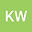An eigenvalue problem for nonlinear Schrödinger-Poisson system with steep potential well
•• Kuan-Hsiang Wang
Kuan-Hsiang Wang
National University of Kaohsiung
Author Profile## Abstract

In this paper, we study an eigenvalue problem for Schrödinger-Poisson system with indefinite nonlinearity and potential well as follows: -Δu+μV(x)u+K(x)Φu = λf(x)u+g(x)|u|p-2u in R3, -ΔΦ=K(x)u^2 in R3, where 4≤p<6, the parameters μ, λ>0, V∈ C(R3) is a potential well, and the functions f ∈ L3/2(R3) and g ∈ L(R3) are allowed to be sign-changing. It is well known that such a system with the potential being positive constant has two positive solutions when lim|x|→∞g(x)=g<0, K=0 in the set {x ∈ R3 : g(x)=0} and λ>λ1(f) with near λ1(f), where λ1(f) is the first eigenvalue of -Δ+ id in H1(R3) (see e.g. Huang et al., J. Differential Equations 255, 2463 (2013)). The main purpose is to obtain the existence and multiplicity of positive solutions without the above assumptions for g and K. The results are obtained via variational method and steep potential. Furthermore, we also consider the concentration of solutions as μ→∞.

#### Peer review status:Published

2021Published in Communications on Pure & Applied Analysis volume 0 issue 0 on pages 0. 10.3934/cpaa.2021030